Refer to our Texas Go Math Grade 4 Answer Key Pdf to score good marks in the exams. Test yourself by practicing the problems from Texas Go Math Grade 4 Lesson 13.4 Answer Key Classify Quadrilaterals.

Essential Question

How can you sort and classify quadrilaterals?
A quadrilateral has four sides and four vertices. The rectangle is a quadrilateral with four right angles. A Rhombus is a quadrilateral with four congruent sides. A Trapezoid is a quadrilateral with exactly one pair of parallel opposite sides.

Unlock the Problem

A quadrilateral is a polygon with four sides and four angles. You can name a quadrilateral by the vertices of its angles.

Quadrilateral ABCD is a possible name for the figure shown at the right. Quadrilateral ACBD is not a possible name, since points A and C are not endpoints of the same side.

Assume that line segments that appear to be parallel are parallel.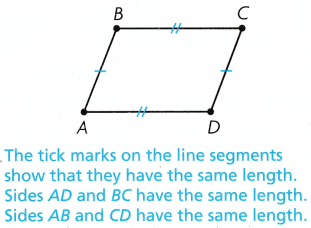Activity 1 Identify right angles in quadrilaterals.

Materials

• color pencils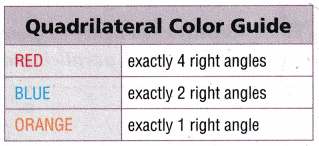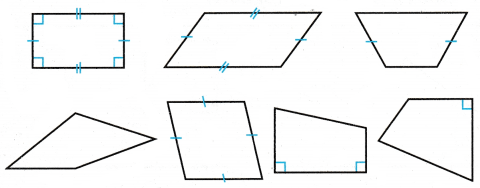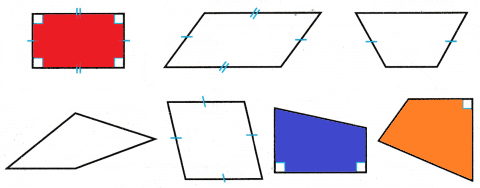Math Talk

Mathematical Processes
Can a quadrilateral have exactly 3 right angles? Explain.
Quadrilaterals have 4 sides and 4 angles. That is no interior angle is less than 180 degrees that add up to 360 degrees ( 4 right angles). Therefore, if 3 internal angles are right angles, the 4th angle must also be a right angle. Hence no quadrilaterals have exactly 3 right angles.

Activity 2 Use a Venn diagram to sort quadrilaterals.

Write the names of the quadrilaterals in the Venn diagram.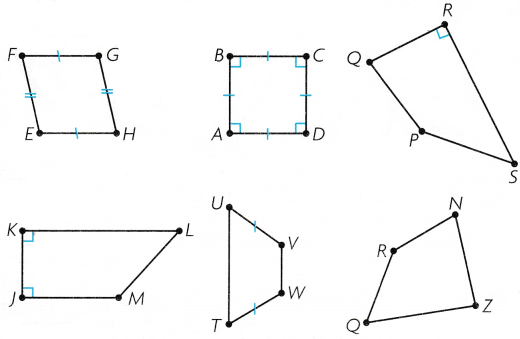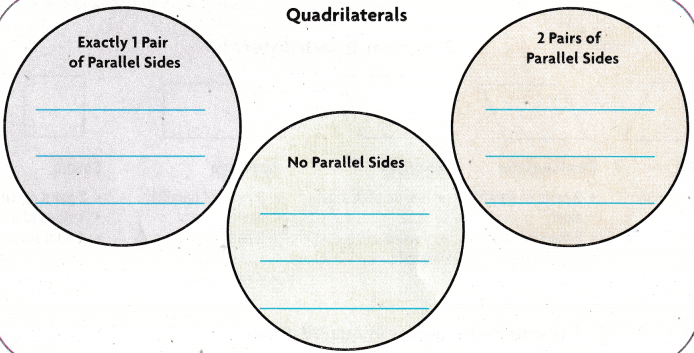Try This Classify each figure as many ways as possible. Write quadrilateral, trapezoid, parallelogram, rhombus, rectangle, or square.

a.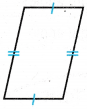Rhombus

Explanation:
The name of the given figure is a rhombus. It has two pairs of parallel sides and 4 sides of equal length.

b.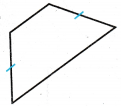Trapezoid

Explanation:
The name of the given figure is a Trapezoid. It has one pair of parallel sides.

c.Square

Explanation:
The name of the given figure is square has two pairs of parallel sides and 4 sides of equal length.

Share and Show

Question 1.
Tell whether the quadrilateral is also a trapezoid, parallelogram, rhombus, rectangle, or square.Quadrilateral ABCD is also a __________________ .
The quadrilateral ABCD is also a trapezoid, parallelogram, rhombus, rectangle, or square

Explanation:
The quadrilateral has 2 pairs of parallel sides, 2 sides of equal length, and has 2 right angles.
In the given figure the Quadrilateral ABCD is a parallelogram that has 2 pairs of parallel sides and 2 sides of equal length.

Classify each figure as many ways as possible. Write quadrilateral, trapezoid, parallelogram, rhombus, rectangle, or square.

Question 2.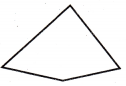Explanation:
The given figure that classifies is Quadrilateral.

Question 3.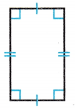Rectangle

Explanation:
The given figure has 2 pairs of parallel sides, 2 pairs of equal length, and 4 right angles. Hence we can name the figure has rectangle.

Question 4.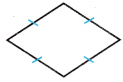Rhombus

Explanation:
The name of the given figure is a rhombus. It has two pairs of parallel sides and 4 sides of equal length.

Math Talk

Mathematical Processes
How would you classify a figure with 4 sides, none of which are parallel? Explain.
The term for such a quadrilateral is called a trapezium. It is a quadrilateral that has 4 sides no parallel sides.

Problem Solving

Question 5.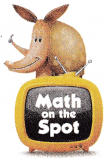H.O.T. Multi-Step Explain how a rhombus and square are alike, and how they are different.
The sides of a square are perpendicular to each other and its diagonals are of equal length. A rhombus is called a quadrilateral in which the opposite sides are parallel and the opposite angles are equal.

Question 6.
H.O.T. Communicate Describe the quadrilaterals you see in the diagram.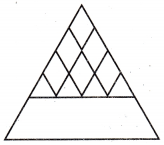Kite, Trapezium

Explanation:
The quadrilaterals that we see in the diagram are kite and Trapezium.

Question 7.
What is the name of the figure shown below?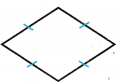(A) rectangle
(B) trapezoid
(C) rhombus
(D) Square
Rhombus

Explanation:
The name of the figure shown below is Rhombus.

Question 8.
Jason wants to classify the figure below in as many ways as possible.Which shows how Jason classifies the figure?
(A) square, trapezoid
(C) rhombus, square, trapezoid

Explanation:
The Jason classifies the figure as Quadrilateral, Trapezoid.

Question 9.
Multi-Step Jane draws two quadrilaterals on construction paper and then cuts them out. One quadrilateral has one more pair of parallel sides than the other quadrilateral. Which two quadrilaterals could Jane have drawn?
(A) rhombus and square
(B) rectangle and trapezoid
(C) rhombus and rectangle
(D) rectangle and square
rectangle and trapezoid

Explanation:
The two quadrilaterals that could jane have been drawn are Rectangle and Trapezoid.

TEXAS Test Prep

Question 10.
Which figure can never have 2 pairs of parallel sides?
(A) trapezoid
(B) rhombus
(C) rectangle
Trapezoid

Explanation:
The figure that can never have 2 pairs of parallel sides is Trapezoid.

### Texas Go Math Grade 4 Lesson 13.4 Homework and Practice Answer Key

Classify each figure as many ways as possible. Write quadrilateral, trapezoid, parallelogram, rhombus, rectangle, or square.

Question 1.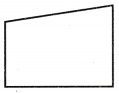Trapezium

Question 2.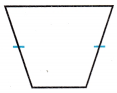Trapezoid

Question 3.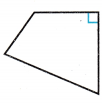Question 4.Rhombus

Question 5.Square

Question 6.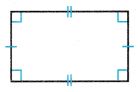Rectangle

Problem Solving

Question 7.
Explain how a trapezoid and a parallelogram are alike, and how they are different.z
A trapezoid has one pair of parallel sides and a parallelogram has two pairs of parallel sides. Therefore we can say that a parallelogram is also a trapezoid. A trapezoid has at least one pair of parallel sides, but it can also have another.

Question 8.
Jake thinks that a rhombus is not always a parallelogram. Is lake correct? Explain.
A parallelogram is a quadrilateral with 2 pairs of parallel sides. The opposite sides on every rhombus are parallel, hence every rhombus is a parallelogram.

Lesson Check

Question 9.
What is the name of the figure shown below?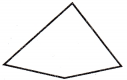(A) triangle
(B) rhombus
(D) parallelogram

Explanation:
The name of the figure that is shown below is Quadrilateral.

Question 10.
Peter wants to classify the figure below in as many ways as possible.Which shows how Peter classifies the figure?
(B) square, parallelogram, rectangle

Explanation:
Peter classifies the figure as Quadrilateral, rectangle, parallelogram.

Question 11.
What is the name of a quadrilateral that always has 4 right angles?
(A) trapezoid
(B) rectangle
(C) rhombus
(D) parallelogram
Rectangle

Explanation:
The name of a quadrilateral that always has 4 right angles is a rectangle.

Question 12.
What is the name of the figure shown below?(A) parallelogram
(B) rhombus
(C) trapezoid
(D) square
Rhombus

Explanation:
The name of the figure shown below is Rhombus.

Question 13.
Multi-Step Chris has 2 short craft sticks of equal length and 2 longer craft sticks of equal length. What are two quadrilaterals he can make with the sticks?
(A) trapezoid and square
(B) rhombus and square
(C) parallelogram and rectangle
(D) trapezoid and rectangle
parallelogram and rectangle

Explanation:
The two quadrilaterals he can make with the sticks are parallelogram and rectangle.

Question 14.
Multi-Step Miranda has four craft sticks of equal length. What are two quadrilaterals she can make with the sticks?
(A) rhombus and square
(B) parallelogram and trapezoid
(C) trapezoid and rectangle
(D) rectangles that are not squares